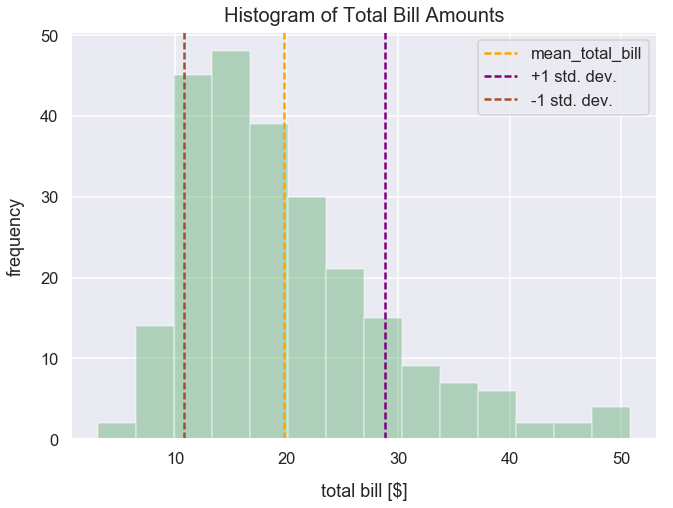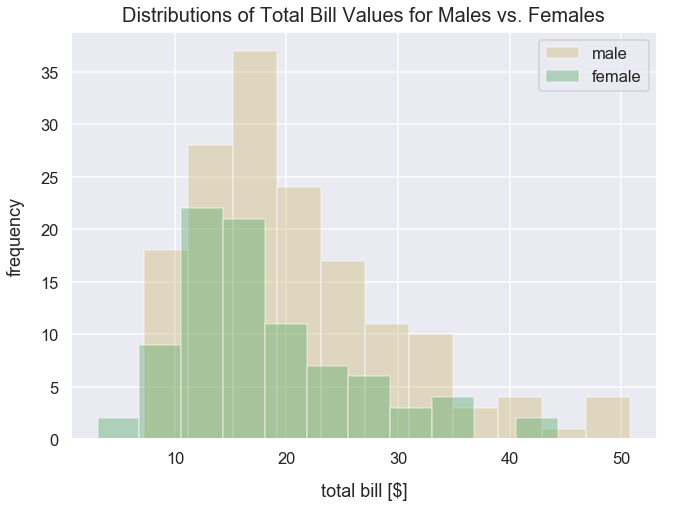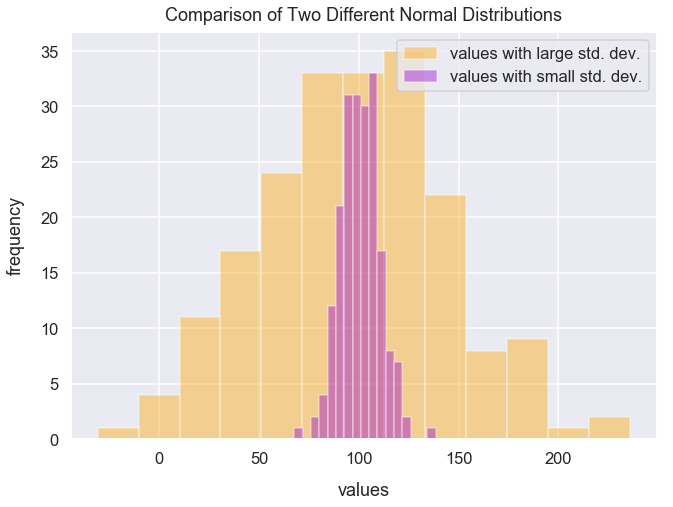Math Descriptive Statistics Article

# Standard Deviation

Standard deviation is a measure of how spread out a set of values are from the mean.

### Sample Vs. Population Standard Deviation¶

Samples tend to underestimate variability of a population. One reason for this is that the values in the sample don't include extremes. Therefore, there are different formulas to calculate a sample and population standard deviation.

Population standard deviation:

$$\sigma = \sqrt{\frac{1}{N}\sum_{i=1}^{N}(x_{i}-\mu)^{2}}$$
• $\sigma$ = population standard deviation
• $N$ = count of values in population
• $x_{i}$ can represent any value in the population
• $\mu$ is the population mean

I'd describe this calculation above as: subtract the mean from each value and calculate its square and you are left with a new list of values. Sum up all those values and you are left with a single number. Lastly, multiple that value by 1 divided by the count of values in the population.

Sample standard deviation:

$$s = \sqrt{\frac{1}{N-1}\sum_{i=1}^{N}(x_{i}-\bar{x})^{2}}$$
• $s$ = sample standard deviation
• $N$ = count of values in sample
• $x_{i}$ can represent any value in the sample
• $\bar{x}$ is the sample mean

In this article, my logic below will just cover analysis to calculate a population standard deviation.

### Import Modules¶

In :
import pandas as pd
import seaborn as sns
import numpy as np
import matplotlib.pyplot as plt
% matplotlib inline


Visualization styling code

In :
sns.set(rc={'figure.figsize':(10.5, 7.5)})
sns.set_context('talk')


### Example 1: Tips Dataset¶

#### Get Tips Data¶

Let's get the tips dataset from the seaborn library and assign it to the DataFrame df_tips.

In :
df_tips = sns.load_dataset('tips')


Each row represents a unique meal at a restaurant for a party of people; the dataset contains the following fields:

column name column description
total_bill financial amount of meal in U.S. dollars
tip financial amount of the meal's tip in U.S. dollars
sex gender of server
smoker boolean to represent if server smokes or not
day day of week
time meal name (Lunch or Dinner)
size count of people eating meal

Preview the first 5 rows of df_tips.

In :
df_tips.head()

Out:
total_bill tip sex smoker day time size
0 16.99 1.01 Female No Sun Dinner 2
1 10.34 1.66 Male No Sun Dinner 3
2 21.01 3.50 Male No Sun Dinner 3
3 23.68 3.31 Male No Sun Dinner 2
4 24.59 3.61 Female No Sun Dinner 4

#### How to Calculate Standard Deviation: The Hard Way on Tips Dataset¶

The value for standard deviation is the square root of the variance. So first, let's calculate variance. We can calculate the variance in the first three steps and the standard deviation in the fourth.

1) Calculate the mean

2) For each value, subtract the mean and square the result (the squared difference)

3) Calculate the average of those squared differences (this is the variance)

4) Calculate the square root of the variance (this is standard deviation)

Let's calculate the standard deviation of our total_bill column in df_tips.

##### 1: Calculate the Mean¶

Use the mean() method in pandas to calculate the mean of the total_bill column in df_tips.

In :
mean_total_bill = round(df_tips['total_bill'].mean(), 2)
mean_total_bill

Out:
19.79
##### 2: Calculate the Squared Differences¶

Create a new column in df_tips that's the difference between each total_bill value and mean_total_bill.

In :
df_tips['total_bill_diff_from_mean'] = df_tips['total_bill'] - mean_total_bill


Preview the first few rows of the columns total_bill and total_bill_diff_from_mean.

In :
df_tips[['total_bill', 'total_bill_diff_from_mean']].head()

Out:
total_bill total_bill_diff_from_mean
0 16.99 -2.80
1 10.34 -9.45
2 21.01 1.22
3 23.68 3.89
4 24.59 4.80

Create a new column called total_bill_squared_differences_from_mean that's the square of total_bill_diff_from_mean.

The square of a value is equivalent to $value*value$; hence the square of 3 is equivalent to $3*3$ which equals 9.

In :
df_tips['total_bill_squared_differences_from_mean'] = df_tips['total_bill_diff_from_mean'].pow(2)


Preview the first few rows of total_bill_squared_differences_from_mean and total_bill_squared_differences.

In :
df_tips[['total_bill_diff_from_mean', 'total_bill_squared_differences_from_mean']].head()

Out:
total_bill_diff_from_mean total_bill_squared_differences
0 -2.80 7.8400
1 -9.45 89.3025
2 1.22 1.4884
3 3.89 15.1321
4 4.80 23.0400
##### 3: Calculate the Variance¶

Calculate the average of values in the total_bill_squared_differences_from_mean column and assign the value to the variable variance_total_bill.

In :
variance_total_bill = round(df_tips['total_bill_squared_differences_from_mean'].mean(), 2)
variance_total_bill

Out:
78.93
##### 4: Calculate the Standard Deviation¶

Use the NumPy sqrt() method to find the square root of variance_total_bill.

In :
standard_deviation_total_bill = round(np.sqrt(variance_total_bill), 0)
standard_deviation_total_bill

Out:
9.0

I can summarize these four steps as the square root of the ((sum of squared deviations divided by n)). I put the parentheses around the latter part to specify an order of operations.

#### How to Calculate Standard Deviation: The Easy Way on Tips Dataset¶

Use the pandas std() method on our total_bill column.

In :
df_tips['total_bill'].std()

Out:
8.902411954856856

#### Visualize Distribution of Total Bill Column¶

Let's visualize the distribution of our total_bill values, the mean, +1 standard deviation, and -1 standard deviation from the mean.

In :
ax = sns.distplot(df_tips['total_bill'], kde=False, color='g')
ax.axes.set_title("Histogram of Total Bill Amounts", fontsize=20, y=1.01)
plt.xlabel("total bill [$]", labelpad=15) plt.axvline(x=mean_total_bill, linestyle='--', linewidth=2.5, label='mean_total_bill', c='orange') plt.axvline(x=(mean_total_bill+standard_deviation_total_bill), linestyle='--', linewidth=2.5, label='+1 std. dev.', c='purple') plt.axvline(x=(mean_total_bill-standard_deviation_total_bill), linestyle='--', linewidth=2.5, label='-1 std. dev.', c='sienna') plt.legend();### Example 2: Tips Dataset Comparing Total Bill Standard Deviation Among Male and Female Servers¶ For each unique value in the sex column, let's see their mean total_bill value and standard deviation of their total_bill values. In : for gender in df_tips['sex'].unique(): std_dev = round(df_tips[df_tips['sex']==gender]['total_bill'].std(), 2) mean = round(df_tips[df_tips['sex']==gender]['total_bill'].mean(), 2) print("Gender {0} has a mean total bill value of {1} and a standard deviation of {2}".format(gender, std_dev, mean))  Gender Female has a mean total bill value of 8.01 and a standard deviation of 18.06 Gender Male has a mean total bill value of 9.25 and a standard deviation of 20.74  Males have a slightly higher standard deviation than females. Below, I plot a histogram of total_bill values to compare males against females. It's slightly noticeable that males have a slightly larger standard deviation than females. In : sns.distplot(df_tips.query("sex=='Male'")['total_bill'], kde=False, color='y', label='male') sns.distplot(df_tips.query("sex=='Female'")['total_bill'], kde=False, color='g', label='female') plt.xlabel("total bill [$]", labelpad=15)
plt.title("Distributions of Total Bill Values for Males vs. Females", y=1.01, fontsize=20)
plt.legend();### Example 3: Compare Vastly Different Standard Deviation Values¶

Using the NumPy package's random module, we can call the normal() method to create a list of values with a normal distribution by setting the following arguments:

• loc as the mean of the distribution
• scale as the standard deviation of the distribution
• size as number of samples
In :
np.random.seed(42) # seed random number generator with fixed value so we always get same values below
high_std_dev_values = list(np.random.normal(loc=100, scale=50, size=200))
low_std_dev_values = list(np.random.normal(loc=100, scale=10, size=200))


Both of the distributions below have a mean of 100.

In :
sns.distplot(high_std_dev_values, kde=False, color='orange', label='values with large std. dev.')
sns.distplot(low_std_dev_values, kde=False, color='darkviolet', label='values with small std. dev.')
plt.title("Comparison of Two Different Normal Distributions", y=1.012)